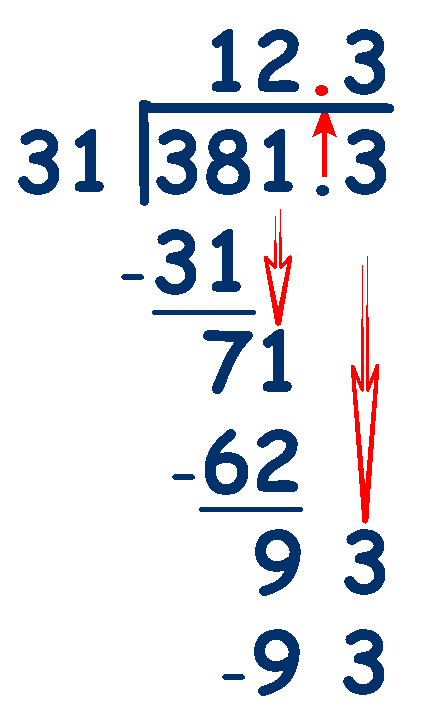# Decimals - Dividing Decimal Numbers

### The best way to divide decimal numbers is to use the Long Division method as shown in Written Methods of Division , but we must remember to account for the position of the decimal points.

If however your child is still using the 'Chunking Method' they need to multiply both numbers by 10 until any decimal numbers are converted into whole numbers. So 7.4 ÷ 3 would become 74 ÷ 30 (7.4 x 10 and 3 x 10) and 8.43 ÷ 2.5 would become 843 ÷ 250 (8.43 x 100 and 2.5 x 100). Then use the 'chunking method' as usual with the whole numbers which will give the correct answer. Notice that you do not need to reverse the effect of multiplying and return the answer to whole numbers and decimal fractions by dividing by the same amount that was used to convert to whole numbers as we did with multiplying. This is becoming very complicated and is made even worse if the number that you are dividing by is larger than the number being divided into. For this reason it is much better to use the Long Division method.

### Using the 'Long Division' method.

If we are dividing a decimal number by a whole number, then we can perform the calculation in the usual way, but ensure that the decimal point is kept in the correct place. ie make sure that the decimal point in the answer is directly above the point in the number that you are dividing. Lets look at an example, 7.6 ÷ 2

(Estimate first - 8 ÷ 2 = 4 - so we know the result should be around 4)

Largest column first - in this case the units.
How many times does 2 go into 7?

3 x 2 = 6 ... put the 3 above the 7

### Remember the decimal point - place it directly above in the answer.

Find the remainder ... 7 -6 = 1 ... put the 1 at the bottom of the units column.

Next, look at the 1/10's column - Bring the 6 down to make 16 the next number to divide into.

How many times does 2 go into 16, 8 x 2 = 16, put 8 above the 6 at the top of the 1/10's column.

There are no remainders so the calculation is complete.

## 7.6 ÷ 2 = 3.8

Check with our estimate of around 4 - OK### But, what if we need to divide a decimal number by a decimal number which has a decimal fraction?

We have to make the number that we are dividing by a whole number and we do that by multiplying by 10 until the number is whole. ie to change 3.14 to a whole number we would multiply by 10, 3.1 x 10 = 31.

Whatever we do to one number, we must do the same to the other. If the number we are dividing is 38.13, then we also multiply that by the same amount, 38.13 x 10 = 381.3

Lets look at an example, 38.13 ÷ 3.1

(Estimate first - 39 ÷ 3 = 13 - so we know the result should be around 13)

## 38.13 ÷ 3.1

First change the number we are dividing by into a whole number ... 3.1 x 10 = 31

Next, change the amount to be divided by the same amount 38.13 x 10 = 381.3

Now calculate 381.3 ÷ 31 as usual

### Remember the decimal point - place it directly above in the answer.

How many 31's go into 38 ... 1 x 31 = 31 .... 38 - 31 = 7

Bring down the 1

How many 31's go into 71 ... 2 x 31 = 62 .... 71 - 62 = 9

Bring down the 3

How many 31's go into 92 ... 3 x 31 = 93 .... 93 - 93 = 0

There are no remainders so the calculation is complete.

## 38.13 ÷ 3.1 = 12.3

Check with our estimate of around 13 - OKTry these worksheets from Maths is Fun